Date Tags skimage

In this post, we’ll see a way to segment nuclei in a confocal microscopy image stack using skimage

First import all we need¶

As I go on with the analysis, I usually add in the first cell all the import statements, rather than having them spread across the notebook. Once development is done, this will go into a script, and those import will already be sorted out.

I’m using the tifffile plugin (see http://www.lfd.uci.edu/~gohlke/code/tifffile.py.html) for the I/O.

In :
import skimage.io as io
io.use_plugin('tifffile')

from skimage.filter import threshold_otsu, threshold_adaptive, rank
from skimage.morphology import label
from skimage.measure import regionprops
from skimage.feature import peak_local_max
from scipy import ndimage
from skimage.morphology import disk, watershed
import pandas as pd

from mpl_toolkits.axes_grid1 import make_axes_locatable
from scipy.spatial import distance as dist
import scipy.cluster.hierarchy as hier

Also, we set the pixel sizes for later. In a wonderfull world, this will be a OME XML file and we would read the metadata carrefully collected by the biologist who acquired the image directely from the file. Of course this is rarely the case.

In :
z_size, x_size, y_size = image_stack.shape

z_scale = 1.5 # µm per plane
xy_scale = 0.71 # µm per pixel

Look at the images¶

In :
nrows = np.int(np.ceil(np.sqrt(z_size)))
ncols = np.int(z_size // nrows + 1)

fig, axes = plt.subplots(nrows, ncols, figsize=(3*ncols, 3*nrows))
for n in range(z_size):
i = n // ncols
j = n % ncols
axes[i, j].imshow(image_stack[n, ...],
interpolation='nearest', cmap='gray')

## Remove empty plots
for ax in axes.ravel():
if not(len(ax.images)):
fig.delaxes(ax)
fig.tight_layout()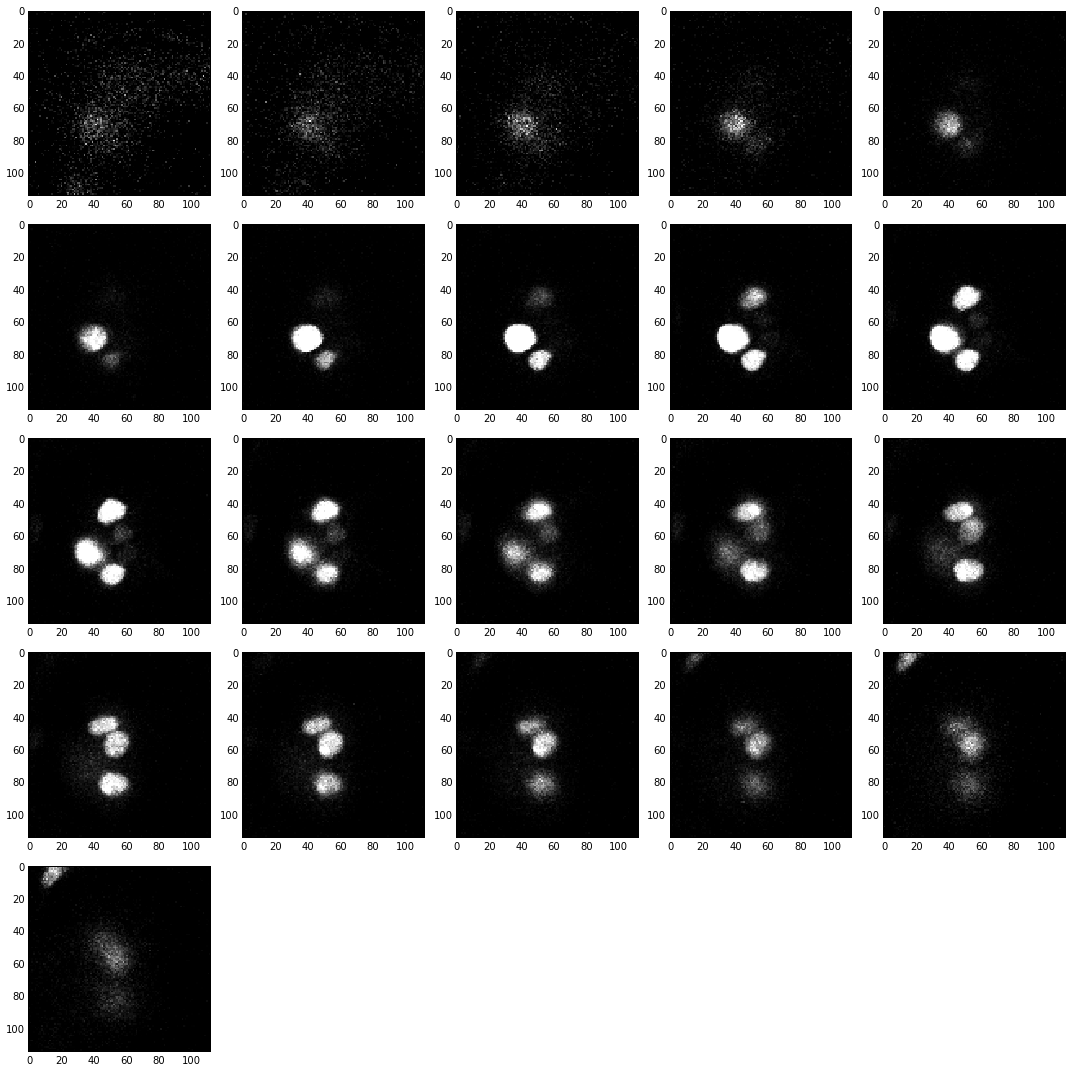In :
fig, ax = plt.subplots(figsize=(8, 4))

ax.hist(image_stack.flatten(), log=True,
bins=4096, range=(0, 4096))

_ = ax.set_title('Min value: %i \n'
'Max value: %i \n'
'Image shape: %s \n'
% (image_stack.min(),
image_stack.max(),
image_stack.shape))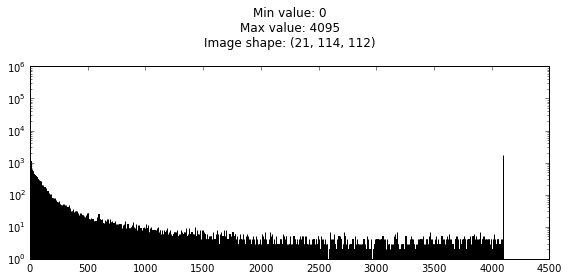Some brief comments on the histogram above. We see that the full 12 bits dynamic range is occupied, which is good. Yet, the much higher peak at the maximum value (4095) tells us some of the signal is saturated, which is not so good, we’ll see why later on. Lastly, the background doesn’t look like the expected background noise for fluorescence imagery, which should follow a Poisson distribution: $P(I) = I / \sigma_I^2 \exp{-I^2/\sigma_I^2} dI$, like the left graph bellow. What we see in the above is the result of an automated background correction, like in the roght graph bellow. So we don’t really deal with raw images…

In :
intensities = np.arange(0, 4096)
background = 1000
dI = 1
proba = (intensities/background**2) * np.exp( - intensities**2 / background**2) * dI
proba /= proba.sum()
n_pixels = 512*512
proba *= n_pixels
proba = proba.astype(np.int)

fig, (ax0, ax1) = plt.subplots(1, 2, figsize=(9, 4))
ax0.step(intensities, proba, 'k')
ax0.set_title('Full histogram')
ax0.set_ylabel('Bin count')
ax0.set_xlabel('Intensity')

ax1.step(intensities[background:], proba[background:], 'k')
ax1.set_title('Background corrected histogram')
ax1.set_xlabel('Intensity')

fig.tight_layout()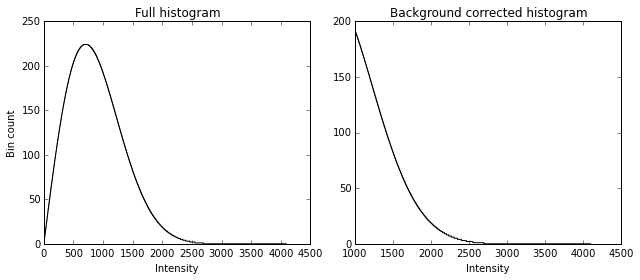Detection parameters¶

We usually need some settings, derived from what we now of the objects we are trying to segment. We’ll also normaly do some kind of smoothing, which will be specified as the pixel size of the filter kernel. So here we go:

In :
smooth_size = 5 # pixels

Overview of the segmentation strategy:¶

In a nutt shell the idea is to (1) threshold, then (2) label the regions above threshold, then (3) segment the labeled regions. We’ll do this on each image plane. After that, we’ll cluster the resulting objects across the $z$ axis

Per plane segmentation¶

Of course this is always the difficult part. Here we use the threshold_otsu function from skimage. We compute an overall threshold over a maximum intensitiy projection along the $z$ axis, and use this threshold to label each stack individually. To ease the segmentation, we filter the image by first smoothing with a median filter and then a local contrast enhancement.

In :
## Computing threshold on the maximum intensity projection with threshold_otsu
max_int_proj = image_stack.max(axis=0)
thresh_global = threshold_otsu(max_int_proj)

smoothed_stack = np.zeros_like(image_stack)
labeled_stack = smoothed_stack.copy()
## Labeling for each z plane:
for z, frame in enumerate(image_stack):
smoothed = rank.median(frame, disk(smooth_size))
smoothed = rank.enhance_contrast(smoothed, disk(smooth_size))
smoothed_stack[z] = smoothed
im_max = smoothed.max()
thresh = thresh_global
# thresh = threshold_otsu(smoothed)
if im_max < thresh_global:
labeled_stack[z] = np.zeros(smoothed.shape, dtype=np.int32)
else:
binary = smoothed > thresh

distance = ndimage.distance_transform_edt(binary)
indices=False, labels=smoothed)
markers = ndimage.label(local_maxi)
/home/guillaume/python3/lib/python3.3/site-packages/scikit_image-0.10dev-py3.3-linux-x86_64.egg/skimage/filter/rank/generic.py:63: UserWarning: Bitdepth of 11 may result in bad rank filter performance due to large number of bins.
"performance due to large number of bins." % bitdepth)

Result of the segmentation¶

In :
fig, axes = plt.subplots(nrows, ncols*2, figsize=(3*ncols, 1.5*nrows))

for z in range(z_size):
i = z // ncols
j = z % ncols * 2
axes[i, j].imshow(smoothed_stack[z, ...], interpolation='nearest', cmap='gray')
axes[i, j+1].imshow(labeled_stack[z, ...], interpolation='nearest', cmap='Dark2')

axes[i, j].set_xticks([])
axes[i, j].set_yticks([])
axes[i, j+1].set_xticks([])
axes[i, j+1].set_yticks([])

## Remove empty plots
for ax in axes.ravel():
if not(len(ax.images)):
fig.delaxes(ax)

fig.tight_layout()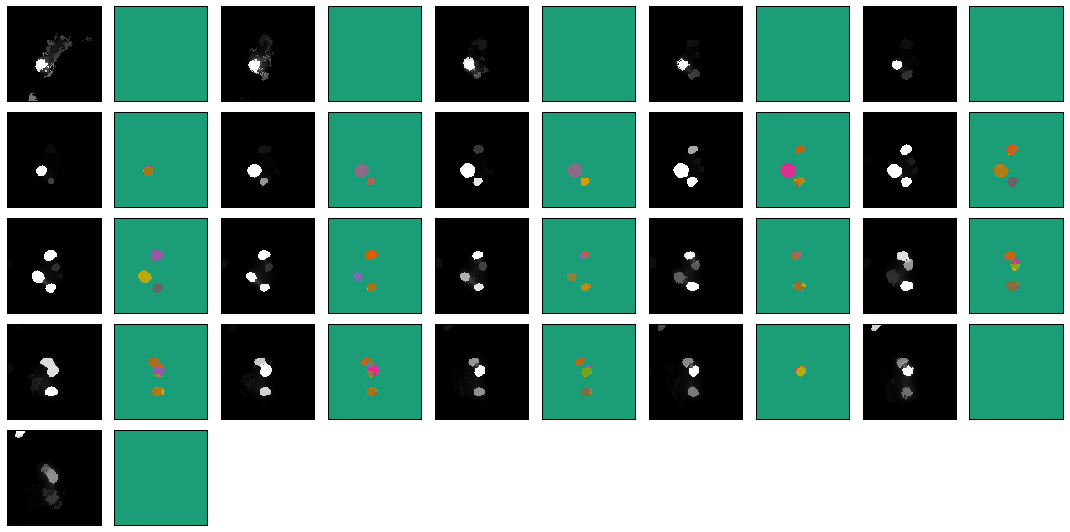a closer look:

In :
fig, axes = plt.subplots(1, 2, figsize=(8, 16))

z = z_size // 2
axes.imshow(smoothed_stack[z, ...], interpolation='nearest', cmap='gray')
axes.imshow(labeled_stack[z, ...], interpolation='nearest', cmap='Dark2')
Out:
<matplotlib.image.AxesImage at 0x7fd55d9b2110>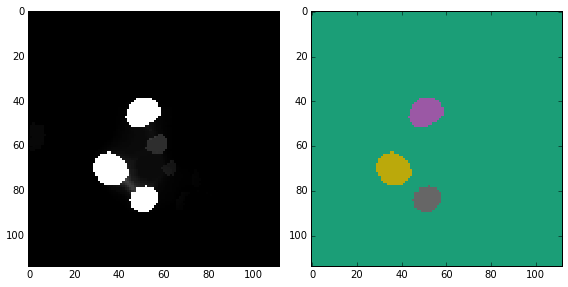Computing the properties of the labeled regions:¶

We use skimage.measure handy function regionprops. For convinience, we store the computed properties in a pandas DataFrame object (this is particullarly usefulll if you have more images or more timepoints, and want to later on manipulate the collected data.

In :
properties = []
columns = ('x', 'y', 'z', 'I', 'w')
indices = []
for z, frame in enumerate(labeled_stack):
f_prop = regionprops(frame.astype(np.int),
intensity_image=image_stack[z])
for d in f_prop:
properties.append([d.weighted_centroid,
d.weighted_centroid,
z, d.mean_intensity * d.area,
indices.append(d.label)
if not len(indices):
all_props = pd.DataFrame([], index=[])
indices = pd.Index(indices, name='label')
properties = pd.DataFrame(properties, index=indices, columns=columns)
properties['I'] /= properties['I'].max()

Here is what we collected:

In :
Out:
x y z I w
label
6 71.249178 40.507531 5 0.394966 5.808687
1 70.186552 39.377994 6 0.854559 8.156394
2 82.987933 50.992160 6 0.171050 4.583498
1 70.022599 38.560650 7 0.990137 8.758578
3 83.256457 50.622169 7 0.316493 5.262410

5 rows × 5 columns

Let’s look at what we collected, plotted over the segmented image

In :
fig, axes = plt.subplots(nrows, ncols, figsize=(3*ncols, 3*nrows))

for z in range(z_size):
plane_props = properties[properties['z'] == z]
if not(plane_props.shape) :
continue
i = z // ncols
j = z % ncols
axes[i, j].imshow(labeled_stack[z, ...],
interpolation='nearest', cmap='Dark2')
axes[i, j].set_xticks([])
axes[i, j].set_yticks([])
x_lim = axes[i, j].get_xlim()
y_lim = axes[i, j].get_ylim()

axes[i, j].scatter(plane_props['y'], plane_props['x'],
s=plane_props['I']*200, alpha=0.4)
axes[i, j].scatter(plane_props['y'], plane_props['x'],
s=40, marker='+', alpha=0.4)
axes[i, j].set_xlim(x_lim)
axes[i, j].set_ylim(y_lim)

## Remove empty plots
for ax in axes.ravel():
if not(len(ax.images)):
fig.delaxes(ax)

fig.tight_layout()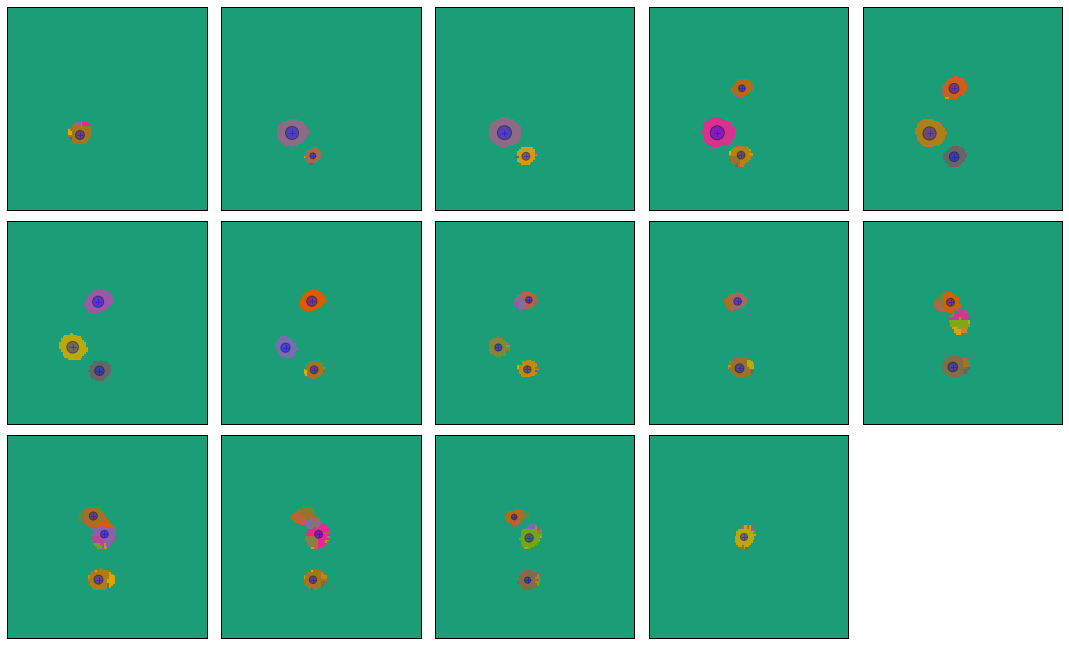Bellow is another way to look at the segmented nuclei positions, this time over intensity projections of the original image.

In :
fig = plt.figure(figsize=(12, 12))
colors = plt.cm.jet(properties.index.astype(np.int32))

# xy projection:
ax_xy.imshow(image_stack.max(axis=0), cmap='gray')
ax_xy.scatter(properties['y'],
properties['x'], c=colors, alpha=1)

divider = make_axes_locatable(ax_xy)
ax_zx = divider.append_axes("top", 2, pad=0.2, sharex=ax_xy)
ax_zx.imshow(image_stack.max(axis=1), aspect=z_scale/xy_scale, cmap='gray')
ax_zx.scatter(properties['y'],
properties['z'], c=colors, alpha=1)
ax_yz = divider.append_axes("right", 2, pad=0.2, sharey=ax_xy)
ax_yz.imshow(image_stack.max(axis=2).T, aspect=xy_scale/z_scale, cmap='gray')
ax_yz.scatter(properties['z'],
properties['x'], c=colors, alpha=1)
plt.draw()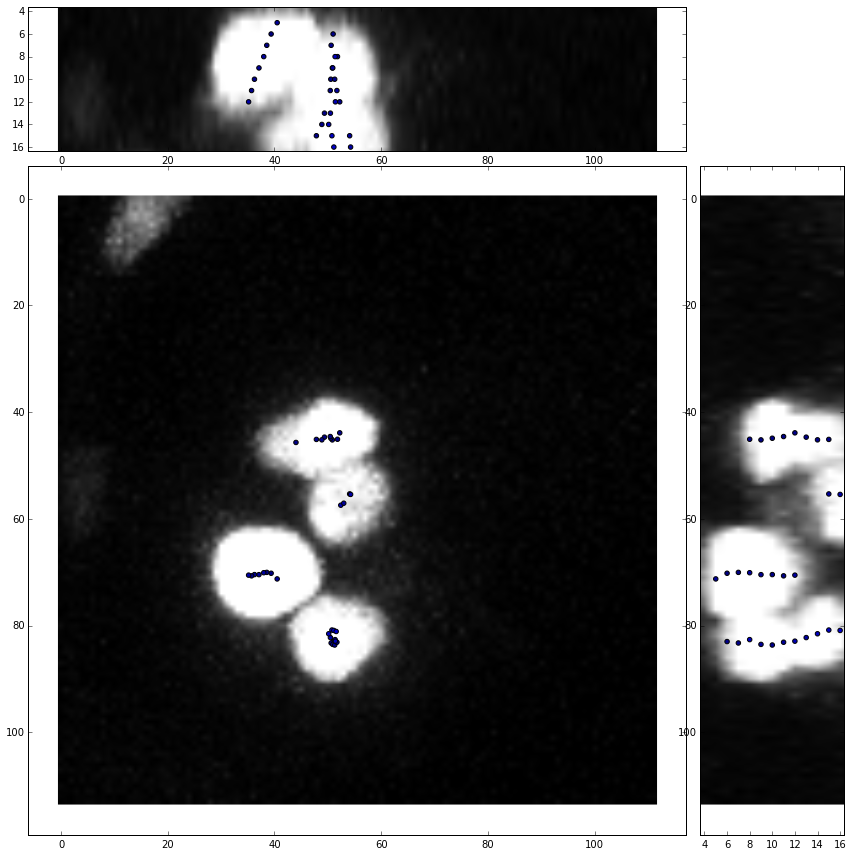Clustering¶

Now that we have detected the nuclei in each plane, we want to regroup them across $z$ so that we have only one 3D position for each of the 4 (or is it 5?) nuclei.

We do so by applying a hierarchical clustering whith scipy.cluster module of the positions in the $(x, y)$ plane. As a parameter for the clustering, we quite naturally use the maximum radius we defined earlier.

In :
positions = properties[['x', 'y']].copy()

dist_mat = dist.squareform(dist.pdist(positions.values))
criterion='distance')
properties['new_label'] = cluster_idx
properties.set_index('new_label', drop=True, append=False, inplace=True)
properties.index.name = 'label'
properties = properties.sort_index()

Now the detected elements are regrouped by label:

In :
Out:
x y z I w
label
1 57.060095 52.985963 18 0.255932 5.556623
1 57.433341 52.431252 17 0.357211 5.698035
1 55.415167 54.268103 16 0.301234 5.232079
1 55.324279 54.091615 15 0.297468 5.382026
2 44.683366 49.372282 13 0.290805 4.982787

5 rows × 5 columns

Next we want to get the center of each cluster. To do so we run a weighted average of the positions for each cluster, using the measured intensity as weight.

In :
def df_average(df, weights_column):
'''Computes the average on each columns of a dataframe, weighted
by the values of the column weight_columns.

Parameters:
-----------
df: a pandas DataFrame instance
weights_column: a string, the column name of the weights column

Returns:
--------

values: pandas DataFrame instance with the same column names as df
with the weighted average value of the column
'''

values = df.copy().iloc
norm = df[weights_column].sum()
for col in df.columns:
try:
v = (df[col] * df[weights_column]).sum() / norm
except TypeError:
v = df[col].iloc
values[col] = v
return values
In :
cell_positions = properties.groupby(level='label').apply(df_average, 'I')

So here is what we where looking for:

In :
cell_positions
Out:
x y z I w
label
1 56.335143 53.412561 16.471683 0.307242 5.474775
2 44.899683 49.921097 11.522186 0.419746 5.945169
3 82.425622 50.970181 11.551490 0.364361 5.626174
4 70.343025 37.816205 8.129519 0.794513 7.884338

4 rows × 5 columns

The final result¶

Let’s plot all that

In :
fig = plt.figure(figsize=(12, 12))
colors = plt.cm.jet(properties.index.astype(np.int32))

# xy projection:
ax_xy.imshow(image_stack.max(axis=0), cmap='gray')
ax_xy.scatter(properties['y'],
properties['x'],
c=colors, alpha=0.2)

ax_xy.scatter(cell_positions['y'],
cell_positions['x'],
c='r', s=50, alpha=1.)

divider = make_axes_locatable(ax_xy)
ax_yz = divider.append_axes("top", 2, pad=0.2, sharex=ax_xy)
ax_yz.imshow(image_stack.max(axis=1), aspect=z_scale/xy_scale, cmap='gray')
ax_yz.scatter(properties['y'],
properties['z'],
c=colors, alpha=0.2)

ax_yz.scatter(cell_positions['y'],
cell_positions['z'],
c='r', s=50, alpha=1.)

ax_zx = divider.append_axes("right", 2, pad=0.2, sharey=ax_xy)
ax_zx.imshow(image_stack.max(axis=2).T, aspect=xy_scale/z_scale, cmap='gray')
ax_zx.scatter(properties['z'],
properties['x'],
c=colors, alpha=0.2)

ax_zx.scatter(cell_positions['z'],
cell_positions['x'],
c='r', s=50, alpha=1.)

plt.draw()